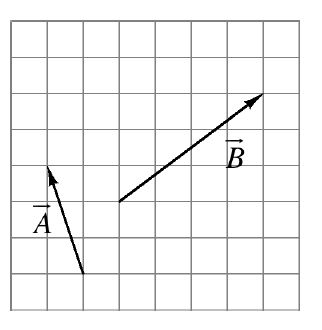### Home > APCALC > Chapter 11 > Lesson 11.1.3 > Problem11-34

11-34.

Use the vectors drawn at right to complete the parts below.

1. Rewrite each vector in both component and $\mathbf{i, j}$ form.

$\mathbf{\vec{A}} = -1\mathbf{i} + 3\mathbf{j}$

2. Determine each vector’s standard angle.

Solve $\tan(θ) = 3/1$. This will solve for the angle in the slope triangle for A.
Recall that the standard angle is measured from the positive $x$-axis.

3. What are $\mathbf{\vec { A }}+\mathbf{\vec { B }}$ and $\mathbf{\vec { B }}-\mathbf{\vec { A }}$ ?

$\mathbf{\vec{A}} + \mathbf{\vec{B}} = 〈 –1, 3〉 + 〈 4, 3〉 = ?$

4. Determine $| | \mathbf{\vec { B } }| | \cdot \mathbf{\vec { A }}$.

The double bars mean "the magnitude of". To calculate the magnitude of vector B, use the Pythagorean Theorem.